2019-12-25 16:11:48 qq_42003997 阅读数 64

# 一个数据集、单个算法

## 一次留出法----二项检验

m个样本的测试集上，泛化错误率为$\epsilon$的学习器被测得测试错误率为$\hat{\epsilon}$的概率是：
$P(\hat{\epsilon} ; \epsilon)=\left(\begin{array}{c} {m} \\ {\hat{c} \times m} \end{array}\right) \epsilon^{\hat{e} \times m}(1-\epsilon)^{m-\hat{e} \times m}$
服从二项分布，可使用“二项检验”进行检验。

## 多次重复留出法或交叉验证法----t检验

多次重复留出法或者交叉验证法进行训练/测试，会得到多个测试错误率，此时可使用“t检验”。假定获得了k个测试错误率，$\hat{\epsilon1}、\hat{\epsilon2}、\hat{\epsilon3}…\hat{\epsilon}k$，则平均测试错误率为$\bar{X}$$S^{2}$
$\bar{X}=\frac{1}{k} \sum_{i=1}^{k} \hat{\varepsilon}_{i} \quad, \quad S^{2}=\frac{1}{k-1} \sum_{i=1}^{k}\left(\hat{\varepsilon}_{i}-\bar{X}\right)^{2}$
根据中心极限定理可知，
$\frac{(\bar{X}-\varepsilon)}{S / \sqrt{n}} \sim t(\mathrm{n}-1)$
t分布示意图及常用双边临界值表如下所示：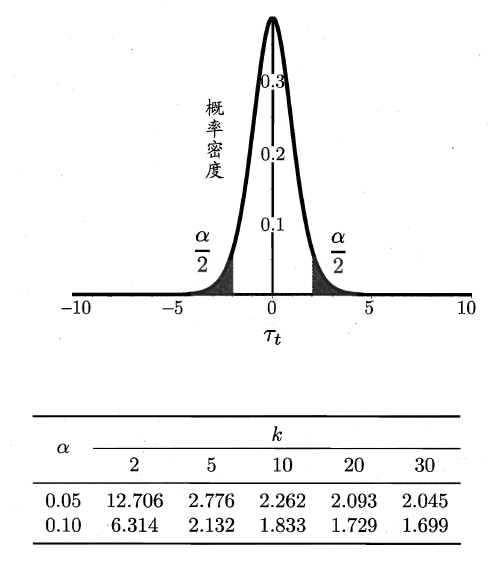# 一个数据集、两个算法----交叉验证t检验

对于一个数据集，两个算法A和B，使用k折交叉验证法得到的测试错误率分别为$\hat{\varepsilon}_{iA} 、 \hat{\varepsilon}_{iB}(i=1,2,,,k)$。可采用k折交叉验证“成对t检验”来进行检验。
基本思想是若两个算法的性能相同，则他们使用相同的训练集/测试集得到的测试错误率应相同，即$\hat{\varepsilon}_{iA} = \hat{\varepsilon}_{iB}$

$\frac{\left|\left(\bar{\Delta}-\left(\varepsilon_{A}-\varepsilon_{B}\right)\right\rangle\right|}{S / \sqrt{\mathrm{k}}} \sim t(\mathrm{k}-1)$
若假设$H_{0}: \varepsilon_{A}=\varepsilon_{B}, H_{1}: \varepsilon_{A} \neq \varepsilon_{B}$，则，满足$\frac{|\bar{\Delta}|}{S / \sqrt{\mathrm{k}}} \leq \mathrm{t}_{\partial / 2}$
由于样本有限，在使用交叉验证等方法的时候，会导致不同轮次的训练集有一定程度的重叠，是的测试错误率不独立，导致过高估计假设成立的概率，为缓解这一问题，可采用“5次2折交叉验证”。

# 一组数据集、多个算法----Friedman检验与Nemenyi检验

## Friedman检验

假设有数据集D1、D2、D3、D4，算法A、B、C进行比较。
使用留出法或者交叉验证得到每个算法在每个数据集上的测试结果，然后在每个数据集上根据测试性能由好到坏排序，并赋予序值1,2,…，若算法测试性能相同，则平分序值。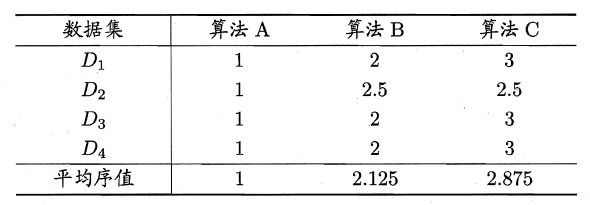使用Friedman检验来判断浙西额算法是否性能相同，若相同，则它们的平均序值应当相同。
若在N个数据集，比较k个算法，令${r_i}$表示第i个算法的平均序值，则${r_i}$的均值和方差分别为$(k+1) / 2, \quad(k+1) \quad(k-1) / 12$，则有：
\begin{aligned} \tau_{\chi^{2}} &=\frac{k-1}{k} \cdot \frac{12 N}{k^{2}-1} \sum_{i=1}^{k}\left(r_{i}-\frac{k+1}{2}\right)^{2} \\ &=\frac{12 N}{k(k+1)}\left(\sum_{i=1}^{k} r_{i}^{2}-\frac{k(k+1)^{2}}{4}\right) \end{aligned}
在k和N比较大时，服从自由度为k-1的$\chi^{2}$分布。
$\tau_{F}=\frac{(N-1) \tau_{\chi^{2}}}{N(k-1)-\tau_{\chi^{2}}}$
服从自由度为k-1和(k-1)(N-1)的F分布。下面是F检验常用的临界值：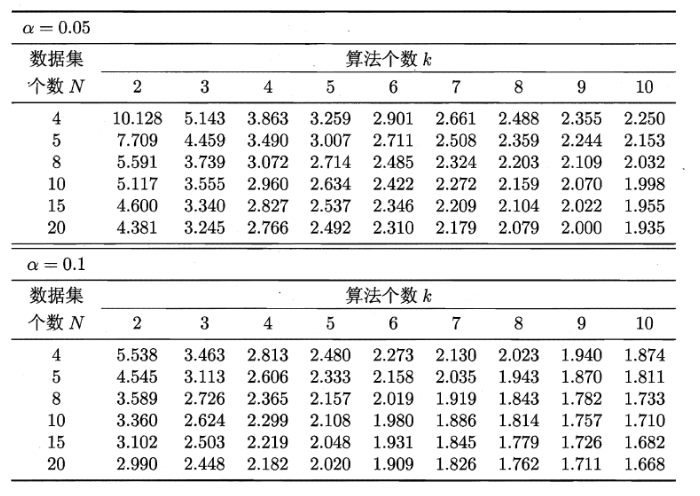## Nemenyi检验

若原假设被拒绝，则需要进行后续检验，来得到具体的算法之间的差异。常用的是Nemenyi检验。Nemenyi检验计算出平均序值差别的临界值域，下表是常用的qa值，若两个算法的平均序值差超出了临界值域CD，则相应的置信度$1-\alpha$拒绝“两个算法性能本相同”的假设。
$C D=q_{\alpha} \sqrt{\frac{k(k+1)}{6 N}}$
qa中常用的联临界值：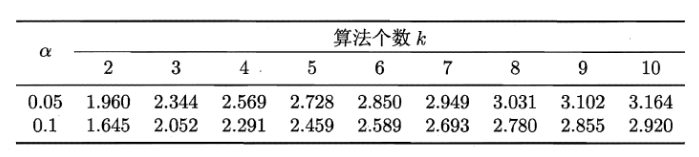# 链接

https://blog.csdn.net/qqMiSa/article/details/98660515

k临界 机器学习实战 相关内容

2019-10-31 11:07:54 yangshengwei230612 阅读数 76

# 1.临界区：

1.临界区：

1.1临界资源

1.2临界区

1.3临界区保护

## 1.1临界资源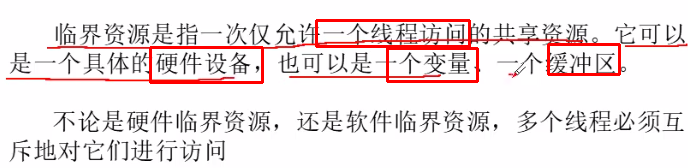## 1.2临界区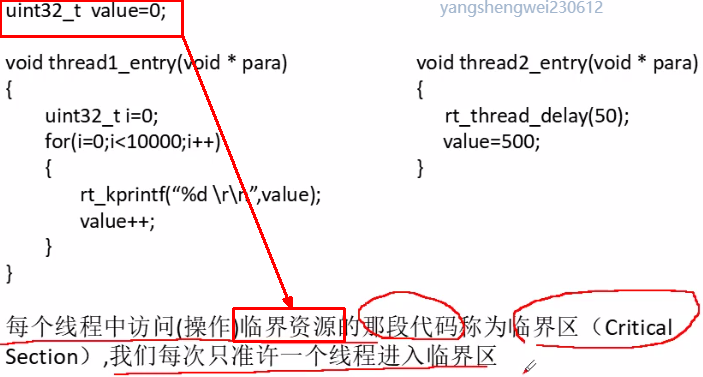## 1.3临界区保护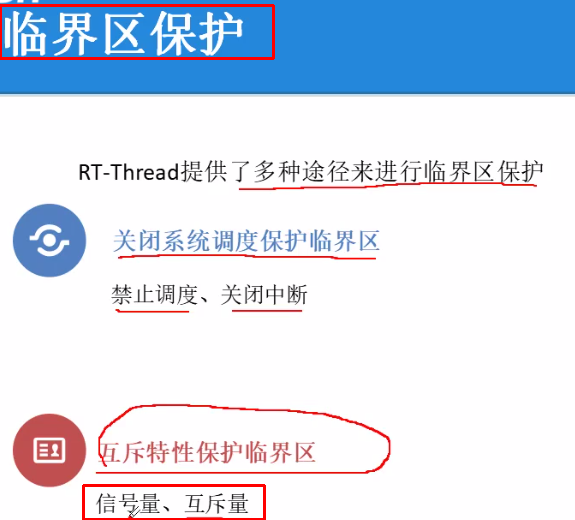### 1.31.关闭系统调度和中断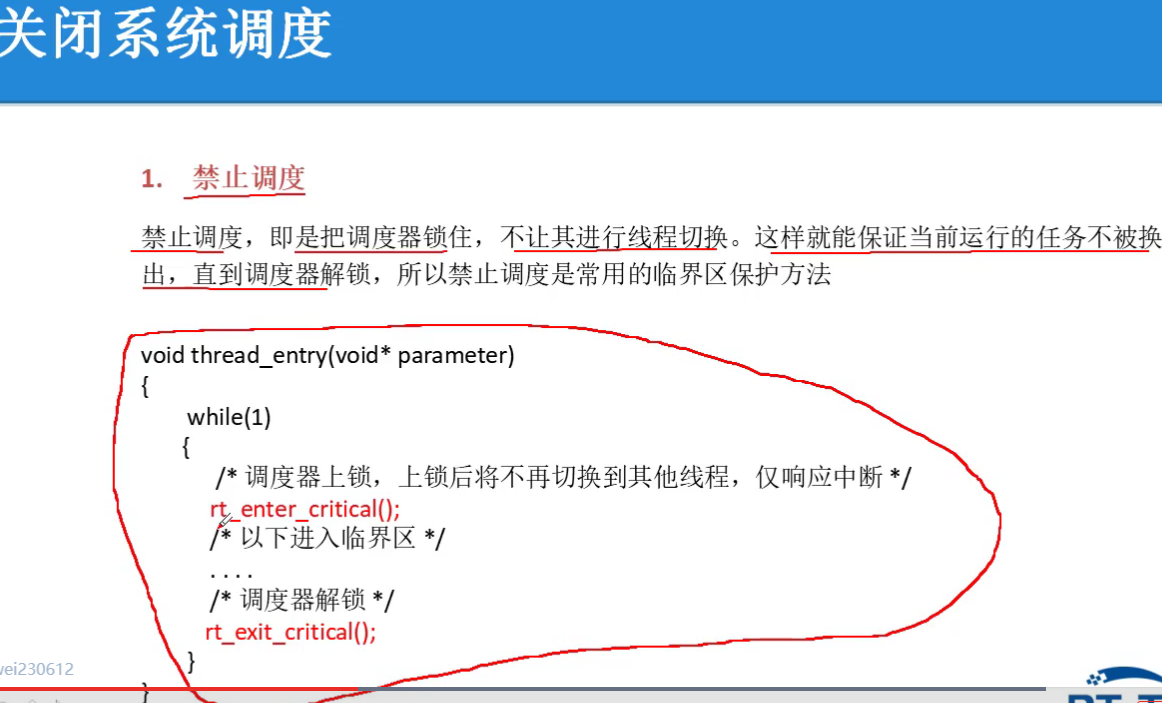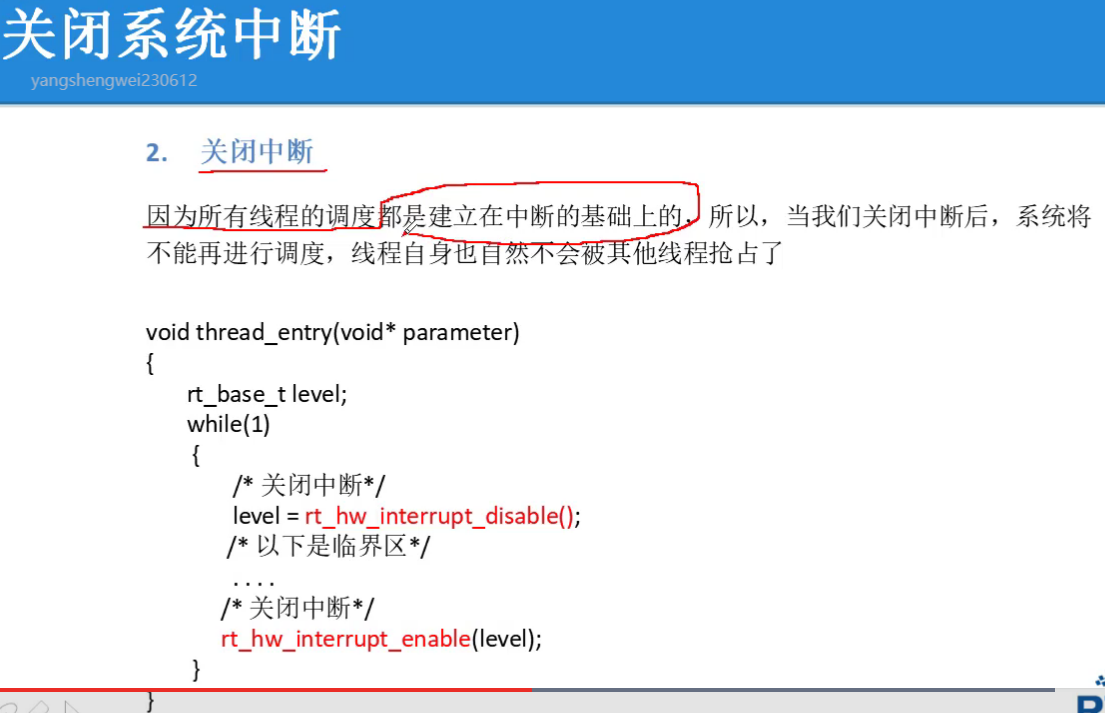k临界 机器学习实战 相关内容

2017-09-01 22:43:19 shiyongraow 阅读数 333

# 处理偏移数据

1）、训练逻辑回归模型hθ(x)。(如果是癌症y=1，否则y=0)
2）、发现你在测试集上有1%的错误率。(99%的诊断是正确的)
3）、只有0.50%的病人患有癌症

function y = predictCancer(x)
y = 0; %ignore x!
return

# 查准率（precision）和召回率（recall）1）、如果有一个样本它实际所属的类是1，预测的类也是1，那么我们把这个样本叫做真阳性（true positive），意思是说我们的学习算法 预测这个值为阳性，实际上这个样本也确实是阳性。
2）、如果我们的学习算法预测某个值是阴性(等于0)，实际的类也确实属于0，那么我们把这个叫做真阴性（true negative），我们预测为0的值实际上也等于0。
3）、如果我们的学习算法预测某个值等于1，但是实际上它等于0，这个叫做假阳性（false positive）。比如我们的算法预测某些病人患有癌症，但是事实上他们并没有得癌症。
4）、最后如果我们的学习算法预测某个值等于0，但是实际上它等于1，这个叫做假阴性（false negative）。因为我们的算法预测值为0，但是实际值是1。

# 权衡精确度和召回率## 权衡不同算法的查准率和召回率（F值）# 机器学习的数据For breakfast I ate __ eggs.

k临界 机器学习实战 相关内容

2011-06-27 17:34:00 blueking109 阅读数 396

多个线程中涉及到同一个临界资源的临界区称为相关临界区。 　　线程进入临界区的调度原则是： ①如果有若干线程要求进入空闲的临界区，一次仅允许一个线程进入。②任何时候，处于临界区内的线程不可多于一个。如已有线程进入自己的临界区，则其它所有 试图进入临界区的线程必须等待。③进入临界区的线程要在有限时间内退出，以便其它线程能及时进入自己的临界区。④如果线程不能进入自己的临界区，则应让出 CPU，避免线程出现“忙等”现象。 　　如果有多个线程试图同时访问临界区，那么在有一个线程进入后其他所有试图访问此临界区的线程将被挂起，并一直持续到进入临界区的线程离开。临界区在被释放后，其他线程可以继续抢占，并以此达到用原子方式操作共享资源的目的。

----摘自百度百科

1.工作中遇到的问题: 同一个类A中有多处同时使用同一个临界区, 即会出现如下情况时

FuncA()

| enter---------------FuncB----------------leave

FuncB()

|-----------enter-----------------------------------------leave

如果是一个线程调用FuncA后,另一个线程调用FuncB 则此时发生资源互斥,导致调用FuncB的线程无期限等待,造成未响应

但如果是同一个线程调用FuncA后 又调用FucnB 则正常执行

解绝外部线程调用FuncB的方法

使用windows msg: SendMessage,PostMessage,  在类A的消息处理函数中调用FuncB

k临界 机器学习实战 相关内容

2017-06-15 09:11:53 swee111 阅读数 162

uC/OS-III 定义了一个进入临界段的宏和两个出临界段的宏。

OS_CRITICAL_ENTER（），

OS_CRITICAL_EXIT（），

OS_CRITICAL_EXIT_NO_SCHED（）

1.在上面我们说过的，当OS_CFG_ISR_POST_DEFERRED_EN 被设为0，在进入临界段之前uC/OS-III 会关中断，在离开临界段之后开中断。OS_CRITICAL_ENTER() 调用uC/CPU 的宏CPU_CRITICAL_ENTER() ， 然后调用CPU_SR_Save() 。CPU_SR_Save()是用汇编写的用于保存当前CPU 寄存器并关中断。寄存器值以类型为“cpu_sr”的变量存于调用者堆栈。

OS_CRITICAL_EXIT() 和OS_CRITICAL_EXIT_NO_SCHED() 都会调用uC/CPU 的宏CPU_CRITICAL_EXIT() 。CPU_CRITICAL_EXIT()调用CPU_SR_Restore()。CPU_SR_Restore（）恢复所保存寄存器值到CPU 寄存器，也就是OS_CRITICAL_ENTER()调用前的状态。如下：

#define  OS_CRITICAL_ENTER()                    CPU_CRITICAL_ENTER()
#define  OS_CRITICAL_ENTER_CPU_EXIT()
#define  OS_CRITICAL_EXIT()                     CPU_CRITICAL_EXIT()
#define  OS_CRITICAL_EXIT_NO_SCHED()            CPU_CRITICAL_EXIT()

2.测量关中断的时间

uC/CPU 提供了测量关中断时间的功能。通过设置CPU_CFG.H 中的CPU_CFG_TIME_MEAS_INT_DIS_EN （CPU_configure_time_measure_intrrupt_distance_enable其实是很好记的）为1 启用该功能。每次关中断前开始测量，开中断后结束测量。每个任务的关中断时间在上文保存的时候被保存于OS_TCB（详见OS_CPU.C 中的OSTaskSwHook()和第八章"上下文切换"）

1.当设置OS_CFG_ISR_POST_DEFERRED_EN 为1 时，在进入临界段前uC/OS-III 会锁住调度器，退出临界段后开启调度器。OS_CRITICAL_ENTER()递增OSSchedLockNestingCtr，给调度器加锁。这是一个决定调度器是否被开启的变量。如果它不为0 则调度器被锁。{称它为调度器锁嵌套值，表示调度器被加了几把锁}

OS_CRITICAL_EXIT()将OSSchedLockNestingCtr 递减，给调度器解锁。{调度器锁嵌套值被减为0 时，就会调用调度器}
OS_CRITICAL_EXIT_NO_SCHED() 也递减OSSchedLockNestingCtr 的值，不同的是当其值减为0 时，不调用调度器。

2.测量锁调度器时间uC/OS-III 提供了测量锁调度器时间的功能，通过设置OS_CFG.H中的OS_CFG_SCHED_LOCK_TIME_MEAS_EN 为1 开启。加锁调度器前测量开始，解锁调度器后测量结束。测得的两种值为:总的锁调度器时间，每个任务的锁调度器时间。因此，用户可以知道每个任务的锁调度器时间，并根据此优化代码。

uC/OS-III 中会用到临界段， 用关中断(OS_CFG.H 中设置OS_CFG_ISR_POST_DEFERRED_EN 为0) 或者锁调度器（ 设置OS_CFG_ISR_POST_DEFERRED_EN 为1）实现保护临界段的功能。用户程序不能使用这些代码：
OS_CRITICAL_ENTER()
OS_CRITICAL_EXIT()
OS_CRITICAL_EXIT_NO_SCHED()

k临界 机器学习实战 相关内容